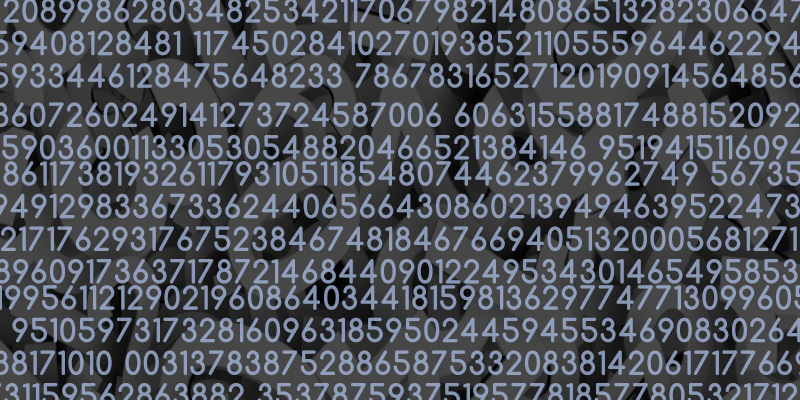# Working with large numbers [Android]A couple of years ago I developed an app that was click based with text and what you had to do was to collect as much money as possible in order to buy new buildings and things like that. What I didn’t think of (then) was that some time since you get such a big income the Integer wouldn’t be enough. Integer’s range starts from -2147483648 and goes up to 2147483647. This is because it is 32bit value, so it is 231. When you reach the maximum value this will take you back to minimum value, so money from 2 billions become -2 billions. In order to fix this I had to use a value that has really larger limit.

Variable Min. Value Max. Value
Boolean 0 1
Byte -128 127
Short -32.768 32.767
Char 0 65535
Integer -2.147.483.648 2.147.483.647
Long -9.223.372.036.854.775.808 9.223.372.036.854.775.807
Float 1.4E-45 3.4028235E38
Double 4.9E-324 1.7976931348623157E308

So I decided to work with Double since it would be easier for me to display large numbers using E. I built a dummy project with 1 EditText to have an input, 1 Button and one TextView to see the result. So it looks something like this.I won’t analyse the code behind the buttons or the EditText I will go straight to the formatting part. What I wanted to achieve is to have a number that if is smaller than 10.000.000 to appear normal and if it is larger than that to appear in form of E. Normally the Double would print 7 or 8 digits in front of the E but I only wanted to so I created a Method in order to format the numbers a bit. This method is called NumFormat and it takes a Double number as input and returns a String.

```private String NumFormat(Double number) {
double compare;
String st_result;
compare = 10000000;
if (number>compare)
{
DecimalFormat formatter = new DecimalFormat("0.###E0");
st_result = formatter.format(number);
}
else
{
DecimalFormat simpler = new DecimalFormat("###");
st_result = simpler.format(number);
}
return st_result;
}
```

If number is smaller than the value I compare it to, I want is to format it like a normal Interger, without any decimal digits. but if is larger I want it to be formatted with 3 decimal digits in front of the power and the value of E. Now I will call this method in my Activity.

```con.setOnClickListener(new View.OnClickListener() {
@Override
public void onClick(View v) {
inputnum = input.getText().toString();
number = Double.valueOf(inputnum);
res.setText(NumFormat(number));
}
});
```

In order to test it I will use 3 different numbers and see how it will get formatted.